Courses

# Retro (Past 13 Years) JEE Main (Thermodynamics)

## 33 Questions MCQ Test Chemistry for JEE Advanced | Retro (Past 13 Years) JEE Main (Thermodynamics)

Description
This mock test of Retro (Past 13 Years) JEE Main (Thermodynamics) for JEE helps you for every JEE entrance exam. This contains 33 Multiple Choice Questions for JEE Retro (Past 13 Years) JEE Main (Thermodynamics) (mcq) to study with solutions a complete question bank. The solved questions answers in this Retro (Past 13 Years) JEE Main (Thermodynamics) quiz give you a good mix of easy questions and tough questions. JEE students definitely take this Retro (Past 13 Years) JEE Main (Thermodynamics) exercise for a better result in the exam. You can find other Retro (Past 13 Years) JEE Main (Thermodynamics) extra questions, long questions & short questions for JEE on EduRev as well by searching above.
QUESTION: 1

Solution:
QUESTION: 2

Solution:
QUESTION: 3

### The incorrect expression among the following is (AIEEE 2012)

Solution:
QUESTION: 4

The entropy change involved in the isothermal reversible expansion of 2 moles of an ideal gas from a volume of 10 dm3 to a volume of 100 dm3 at 27° C is

(AIEEE 2011)

Solution:
QUESTION: 5

In view of the signs of ΔrG° for the following reactions.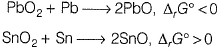Q. Which oxidation states are more characteristic for lead and tin?

(AIEEE 2011)

Solution:
QUESTION: 6

The value of enthalpy change (ΔH) for the reaction,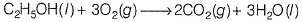at 27°C is - 1366.5 kJ mol-1. The value of internal energy change for the above reaction at th is tem perature will be

(AIEEE 2011)

Solution:
QUESTION: 7

Consider the reaction,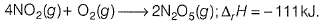If N2O5(s) is formed instead of N2O5(g) in the above reaction, the ΔrH value will be

(Given, ΔH of sublimation for N2O5 is  54 kJ mol-1)

(AIEEE 2011)

Solution:
QUESTION: 8

The standard enthalpy of formation of NH3 is - 46.0 kJ mol-1. If the enthalpy of formation of H2 from its atoms is - 436 kJ mol-1 and that of N2 is - 712 kJ mol-1, the average bond enthalpy of N—H bond in NH3 is

(AIEEE 2010)

Solution:
QUESTION: 9

For a particular reversible reaction, at temperature T, ΔH and ΔS were found to be both +ve. If Te is the temperature at equilibrium, the reaction would be spontaneous when

(AIEEE 2010)

Solution:
QUESTION: 10

In a fuel cell, methanol is used as fuel and oxygen gas is used as an oxidiser. The reaction is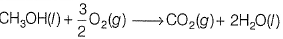at 298 K standard Gibbs' energies of formation for CH3OH(l), H2O(l) and CO2(g) are -166.2, -237.2 and - 394.4 kJ mol-1, respectively. If standard enthalpy of combustion of methanol is -726 kJ mol-1, efficiency of the fuel cell will be

Solution:
QUESTION: 11

On the basis of the following thermochemical data [ΔfG°H+(aq) = 0]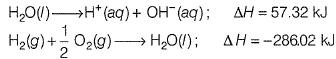Q. The value of enthalpy of formation of OH- ion at 25°C is

(AIEEE 2009)

Solution:
QUESTION: 12

Oxidising power of chlorine in aqueous solution can be determined by the parameters indicated below.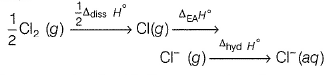The energy involved in the conversion of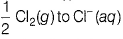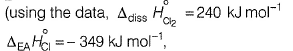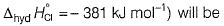(AIEEE 2008)

Solution:
QUESTION: 13

Standard entropy of X2, Y2 and XY3 are 60, 40 and 50 JK-1 mol-1, respectively. For the reaction,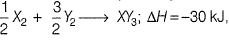to be at equilibrium, the temperature will be

(AIEEE 2008)

Solution:
QUESTION: 14

Assuming that water vapour is an ideal gas, the internal energy change (ΔE) when 1 mole of water is vaporised at 1 bar pressure and 100°C, (Given : molar enthalpy of vaporisation of water at 1 bar and 373 K = 41 kJ mol-1 and R = 8.3 J mol-1 K-1) will be

(AIEEE 2007)

Solution:
QUESTION: 15

Identify the correct statement regarding a spontaneous process.

(AIEEE 2007)

Solution:
QUESTION: 16

In conversion of limestone to lime,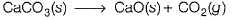the values of ΔH° and ΔS° are +179.1 kJ mol-1 and 160.2 J / K, respectively at 298 K and 1 bar. Assuming that ΔH° and ΔS° do not change with temperature, temperature above which conversion of limestone to lime will be spontaneous it.

(AIEEE 2007)

Solution:
QUESTION: 17

An ideal gas is allowed to expand both reversibly and irreversibly in an isolated system. If Ti is the initial temperature and Tf is the final temperature, then which of the following statements is correct ?

(AIEEE 2006)

Solution:
QUESTION: 18

(ΔH - ΔE) for the formation of carbon monoxide (CO) from its elements at 298 K is

(R - 8.314 JK -1 mol-1)

(AIEEE 2006)

Solution:
QUESTION: 19

The enthalpy changes for the following processes are listed below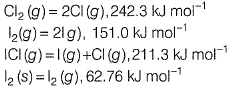Given that the standard states for iodine and chlorine are l2(s)and Cl2(g), the standard enthalpy of formation of ICI (g) is

(AIEEE 2006)

Solution:
QUESTION: 20

The standard enthalpy of formation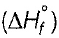at 298 K for methane, CF4 (g) is - 74.8 kJ mol-1. The addition information required to determine the average energy for C—H bond form ation would be

(AIEEE 2006)

Solution:
QUESTION: 21

Consider the reaction,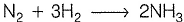carried out at constant temperature and pressure. If ΔH and ΔE are the enthalpy and internal energy changes for the reaction, which of the following expressions is true ?

(AIEEE 2005)

Solution:
QUESTION: 22

If the bond dissociation energies of XY, X2 and Y2 (all diatomic molecules) are in the ratio of 1 : 1 : 0.5 and ΔHf for the formation of XY is -200 kJ mol-1. The bond dissociation energy of X2 Will be

(AIEEE 2005)

Solution:
QUESTION: 23

For a spontaneous reaction, the ΔG, equilibrium constant (K) and E°cell will be respectively

(AIEEE 2005)

Solution:
QUESTION: 24

An ideal gas expands in volume from 1 x 10-3 m 3 to 1 x 10-2 m3 at 300 K against a constant pressure of 1 x 105 Nm-2. The work done is

(AIEEE 2004)

Solution:
QUESTION: 25

The enthalpies of combustion of carbon and carbon monoxide are -393.5 and - 283 kJ mol-1, respectively. The enthalpy of formation of carbon monoxide per mole is

(AIEEE 2004)

Solution:
QUESTION: 26

The enthalpy change for a reaction does not depend upon the

(AIEEE 2003)

Solution:
QUESTION: 27

The internal energy change when a system goes from state A to B is 40 kJ/mol. If the system goes from A to B by a reversible path and returns to state A by an irreversible path, what would be the net change in internal energy?

(AIEEE 2003)

Solution:
QUESTION: 28

If at 298 K, the bond energies of C—H, C—C, C=C and H—H bonds are respectively 414, 347, 615 and 435 kJ mol-1, the value of enthalpy change for the reaction,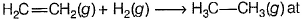298 K will be

(AIEEE 2003)

Solution:
QUESTION: 29

In an irreverible process taking place at constant T and p and in which only pressure-volume work is being done, the change in Gibbs free energy (dG) and change in entropy (dS), satisfy the criteria

(AIEEE 2003)

Solution:
QUESTION: 30

The correct relationship between free energy change in a reaction and the corresponding equilibrium constant Kc is

(AIEEE 2003)

Solution:
QUESTION: 31

Heat required to raise the temperature of 1 mole of a substance by 1° is called

(AIEEE 2002)

Solution:
QUESTION: 32

A heat engine a bsorbs heat q1 from a source at temperature T1 and heat q2 from a source at temperature T2. Work done is found to be J (q1 + q2). This is in accordance with

(AIEEE 2002)

Solution:
QUESTION: 33

A reaction is non-spontaneous at the freezing point of water but is spontaneous at the boiling point of water, then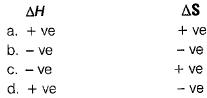(AIEEE 2002)

Solution: